This topic lists the series view types associated with the ASP.NET Chart Control’s Radar and Polar diagram types. It also explains how to access specific options (both at design time and runtime), and briefly describes these options. Before reading this section, you may wish to review the following help topic on diagram fundamentals: Diagram Overview.

The topic consists of the following sections.

## Associated Series View Types

The Radar and Polar diagrams are used to plot Radar and Polar series.

Series of Radar view types (also called Spider or Star charts) display data as a circular graph. A Radar view is drawn on a circular grid, and has multiple axes along which data can be plotted. The following image shows a typical Radar diagram.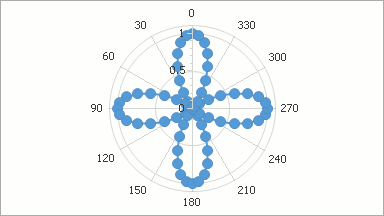Polar view types are similar to Radar views, but display angles (from 0 to 360 degrees) instead of string values or dates on the angular axis. The following image shows a typical Polar diagram.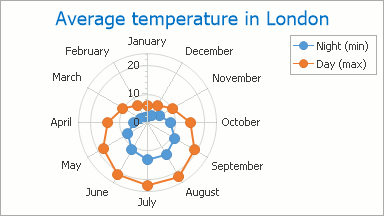The available radar and polar series view types are as follows:

Note that although this chart type normally has a circular shape, it can also be displayed as a polygon.

## Accessing a Diagram

You can access the options of a radar or polar diagram at design time. Click the chart to select it, and in the Properties window, expand the WebChartControl.Diagram property.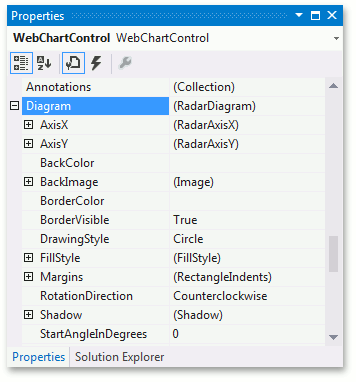At runtime, cast your instance of the Diagram object to the RadarDiagram (or PolarDiagram) type.

``````// Create a new WebChartControl instance.
WebChartControl chart = new WebChartControl();

// Create a series of a compatible view type,
// and add it to the chart's collection,
// so that the diagram object cannot be equal to null.

// Create a diagram, and cast it to the RadarDiagram type.

// Access the diagram's options.
``````

## Specific Options

The PolarDiagram class is derived from the RadarDiagram class, and its properties are identical to the Radar diagram properties. For this reason, this example uses the Radar diagram to demonstrate the available options. For more details on a particular option, refer to the corresponding property description below.

The RadarDiagram.AxisX and the RadarDiagram.AxisY properties allow you to access the radar diagram’s axis options. Note that element implementation for radar and polar axes is different from regular axes. For example, the axis of arguments (X-axis) corresponds to the circular grid onto which Radar series are plotted. You can select this axis by clicking its labels. Refer to the following help topic for more information: Axes.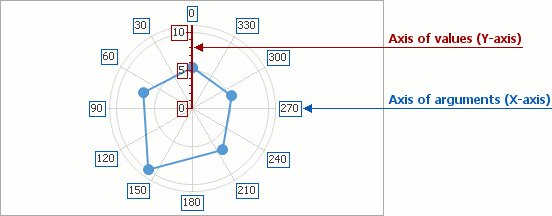The RadarDiagram.DrawingStyle property determines the shape of the grid used to plot a Radar chart (RadarDiagramDrawingStyle.Circle or RadarDiagramDrawingStyle.Polygon). The following images demonstrate this property in action.

DrawingStyle = Circle DrawingStyle = Polygon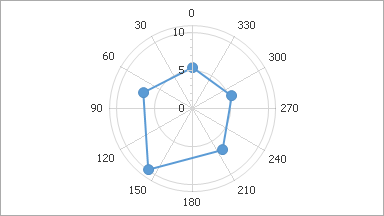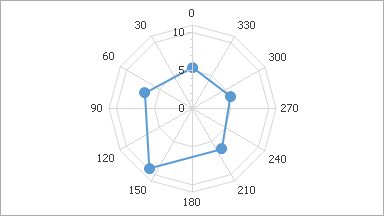The RadarDiagram.RotationDirection property determines the direction in which the diagram’s X-axis displays arguments (clockwise or counterclockwise). The following images demonstrate this property in action.

RotationDirection = Clockwise RotationDirection = Counterclockwise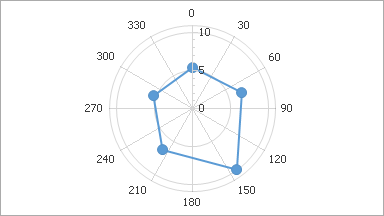The RadarDiagram.StartAngleInDegrees property determines the angle at which to begin drawing the diagram’s X-axis. The following images demonstrate this property in action.

StartAngleInDegrees = 0 StartAngleInDegrees = 60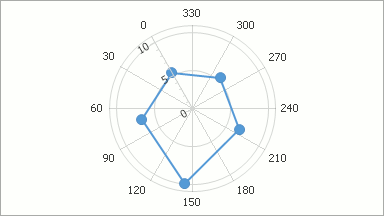Other diagram properties control its appearance in the following ways.